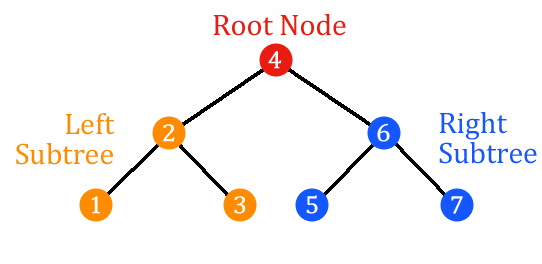# Binary Search Tree

A binary search tree is a data structure that is comprised of nodes in a branching relationship, each node having a key signifying its value. Each node can have zero, one, or two child nodes branching off from it. The tree has one root node that has no parent.

All nodes in the left branch of a parent node have values that are less than the parent node’s value, and all nodes in the right branch of a parent node have values that are greater than the parent node’s value.

Below is a diagram of a binary search tree.The part of the tree with nodes `1`, `2`, and `3` is referred to as the left subtree, and the part with nodes `5`, `6`, and `7` is referred to as the right subtree. Both subtrees are themselves binary search trees. The nodes `1`, `3`, `5`, and `7` are called leaf nodes because they have no children.

## Binary Search Tree vs. Hash Table

Like a hash table, a binary search tree is a method of storing keys for later retrieval and manipulation. Both have their own strengths, and the best storage type to use is dependent on what tasks are going to be undertaken with the data being stored.

If the primary tasks needed are search, insert, or delete, a hash table performs them in O(1) time (in big-O notation). A binary search tree performs them in O(log(n)) time. So if just these operations are all that are needed, a hash table is more performant.

A binary search tree is preferable to a hash table in the following circumstances:

• There’s a need for retrieving keys in sorted order.
• There’s a need for operations like order statistics, finding the next smallest or largest element, or range queries.
• Memory is a consideration. Binary search trees are more memory efficient than hash tables.
• With binary search trees, operations take O(log(n)) time. While hashing averages O(1) time, some operations may be more costly, taking O(n2) time, especially when table resizing happens.

## Binary Search Tree

Inorder Traversal
First traverses the left subtree, then the root, and then the right subtree.
Postorder Traversal
First traverses the left subtree, then the right subtree, and then the root.
Preorder Traversal
First traverses the root, then the left subtree, and then the right subtree.